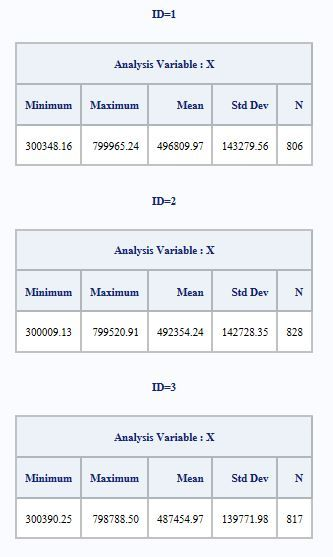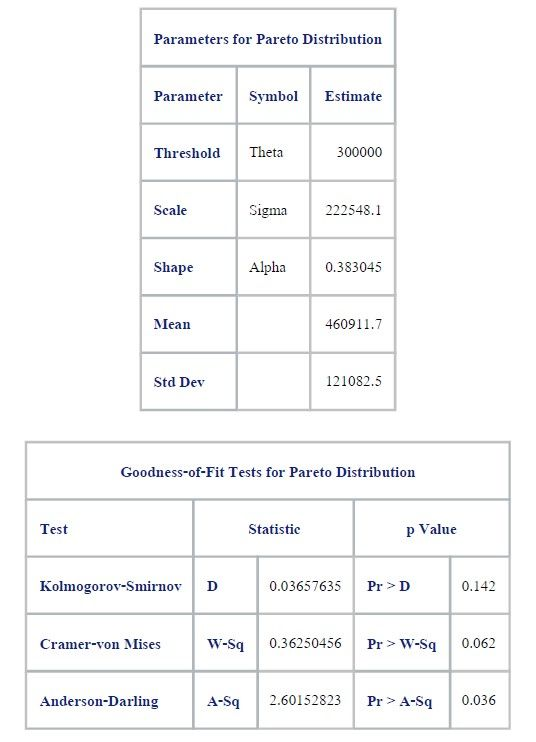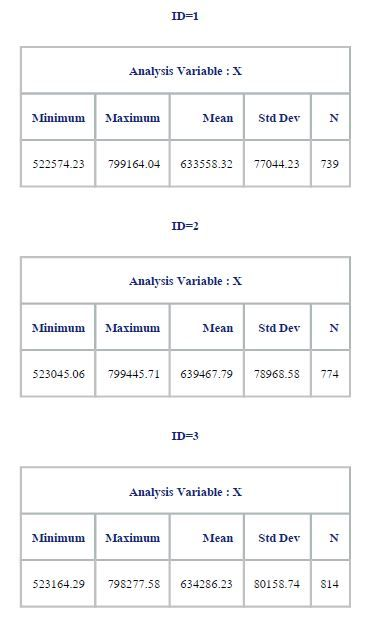## simulating data from gamma and pareto distribution

I am working on modeling data.

By using "Proc univariate" to fit data, distribution that I get is Gamma with theta=60500 and Pareto with theta=300000.

then, I simulate data from these distributions by using code as follow:

%let N=3500;

%let NumSamples = 120;

data simu.pareto1(keep=ID X);

do ID = 1 to &NumSamples;

a = 0.383045;

k = 222548.1;

call streaminit(1234);

do i = 1 to &N;

U = rand("Uniform");

X = k / U**(1/a);

output;

end;

end;

run;

but the output seems deviate from the fitted data quite much.  I'm not sure if it's relevant with the unspecify theta on my code or not?

If yes, please let me know the code for simulation with specify theta (for both Gamma and Pareto).

4 REPLIES 4

## Re: simulating data from gamma and pareto distribution

You can simulate some random variate Y with threshold parameter theta (and possibly a scale parameter sigma, by using the RAND function as you do to create the standard variate X and then create Y = theta + sigma * X.

Your way of simulating a standard Pareto looks good if you want to do this in a datastep, since the RAND function does not support the Pareto distribution. The RANDGEN function in SAS/IML does however support it, so you can do it directly there. The RAND function also supports the Gamma distribution, so simply simulate a Gamma random variate and apply the transformation above.

## Re: simulating data from gamma and pareto distribution

Yes, your Pareto simulation is identical to the one on p. 113 of Simulating Data with SAS.

If you look at pp. 109-111, there is a section on "Adding Location and Scale Parameters."

X = Theta_Pareto + k / U**(1/a);

Similarly for the gamma simulation, use

G = Theta_gamma + rand("gamma", <scale param here>);

You can simulate it in the same DATA step that simulates the Pareto variable.

## Re: simulating data from gamma and pareto distribution

Thank you very much for your kind suggestion above.

However, after I did as per your suggestion, I found out many issues as following:

• First of all, please let me clarify you that the data range of this fitted Pareto distribution is 300,000-800,000 (with theta =300,000). And then I simulated Pareto distribution without identifying theta.
• After I used the coding to add theta as per your suggestion, I think that the output statistic seems worse. Kindly find the compared statistic as below details:

Fitting Pareto distribution -> output1

Simulation without theta -> output2 (after cut off data, so the data range of is 300,000-800,000)

Simulation with theta -> output3 (after cut off data, so the data range of is 300,000-800,000)

As you can see that, the statistic of simulation with theta are different from the fitted Pareto quite much. I am not sure if these output are acceptable or not? If not, please let have your further suggestion.## Re: simulating data from gamma and pareto distribution

1. If you want us to match data, you need to supply some sample data in the form of a SAS DATA step.  It is difficult to guess what difficulties you might be having without a common set of data that everyone can run.

2. Numerically speaking, I would suggest measuring units in thousands, so that your data are 300-800 (with theta =300). This is likely to be more robust.

Discussion stats
• 4 replies
• 2005 views
• 2 likes
• 3 in conversation